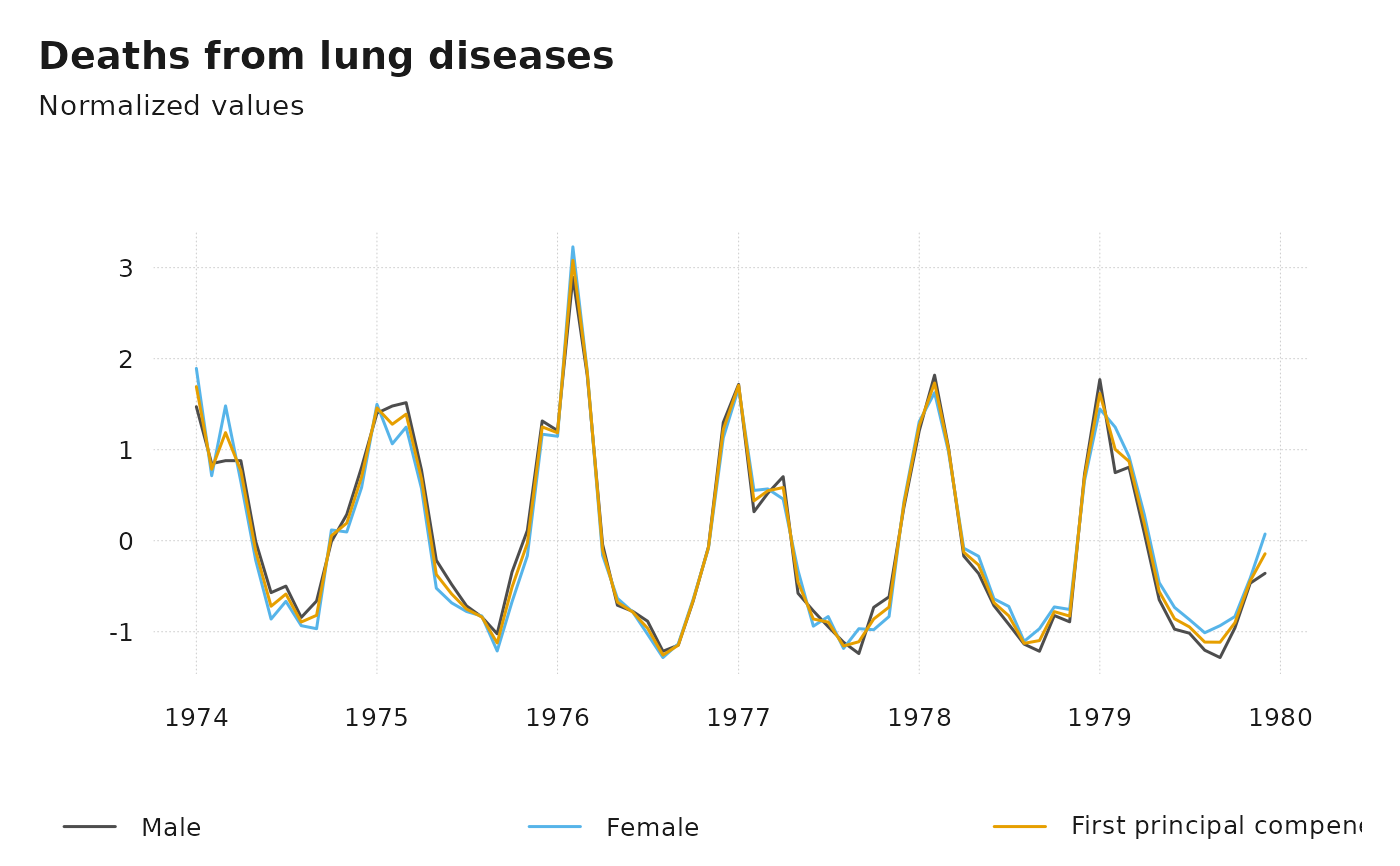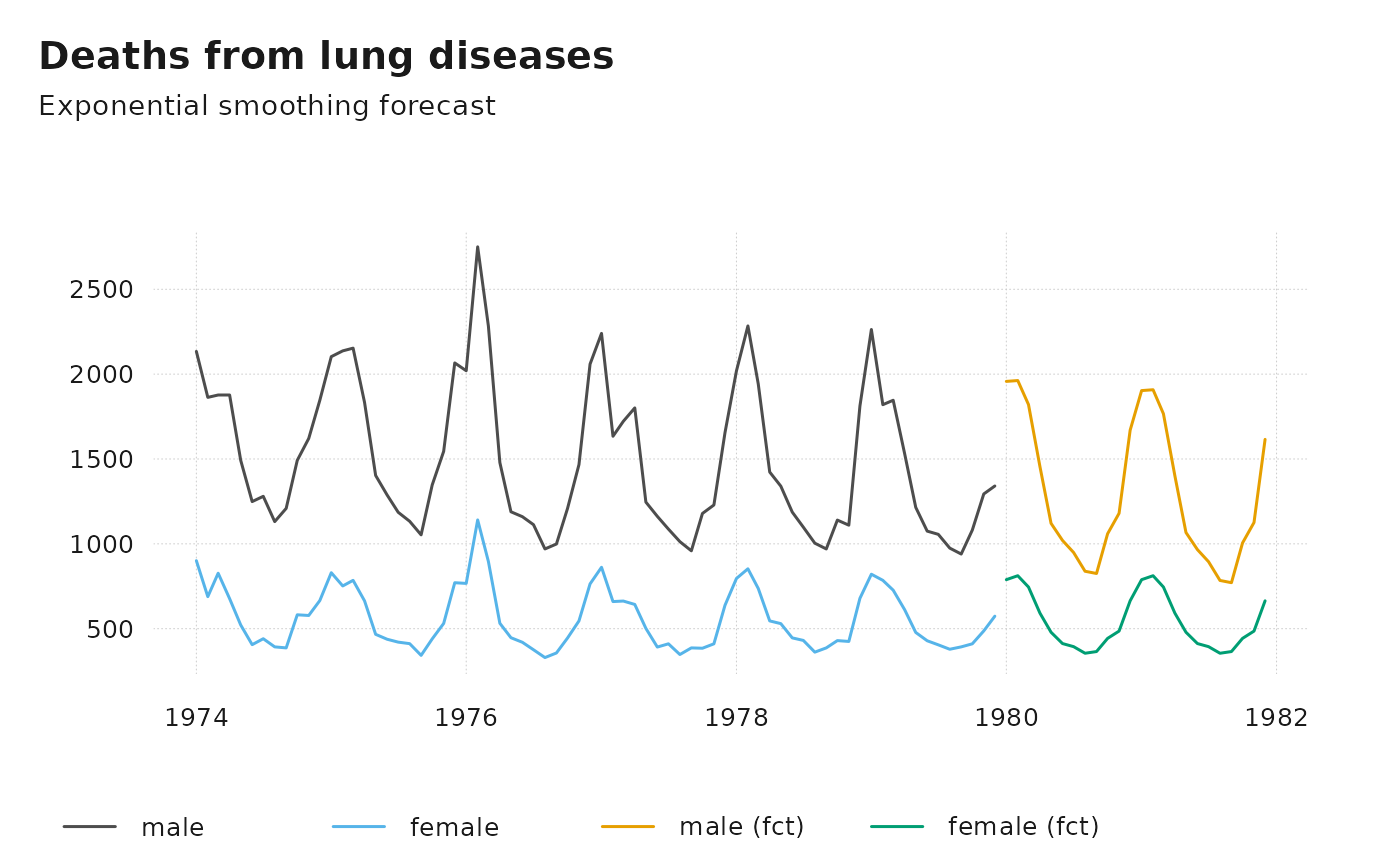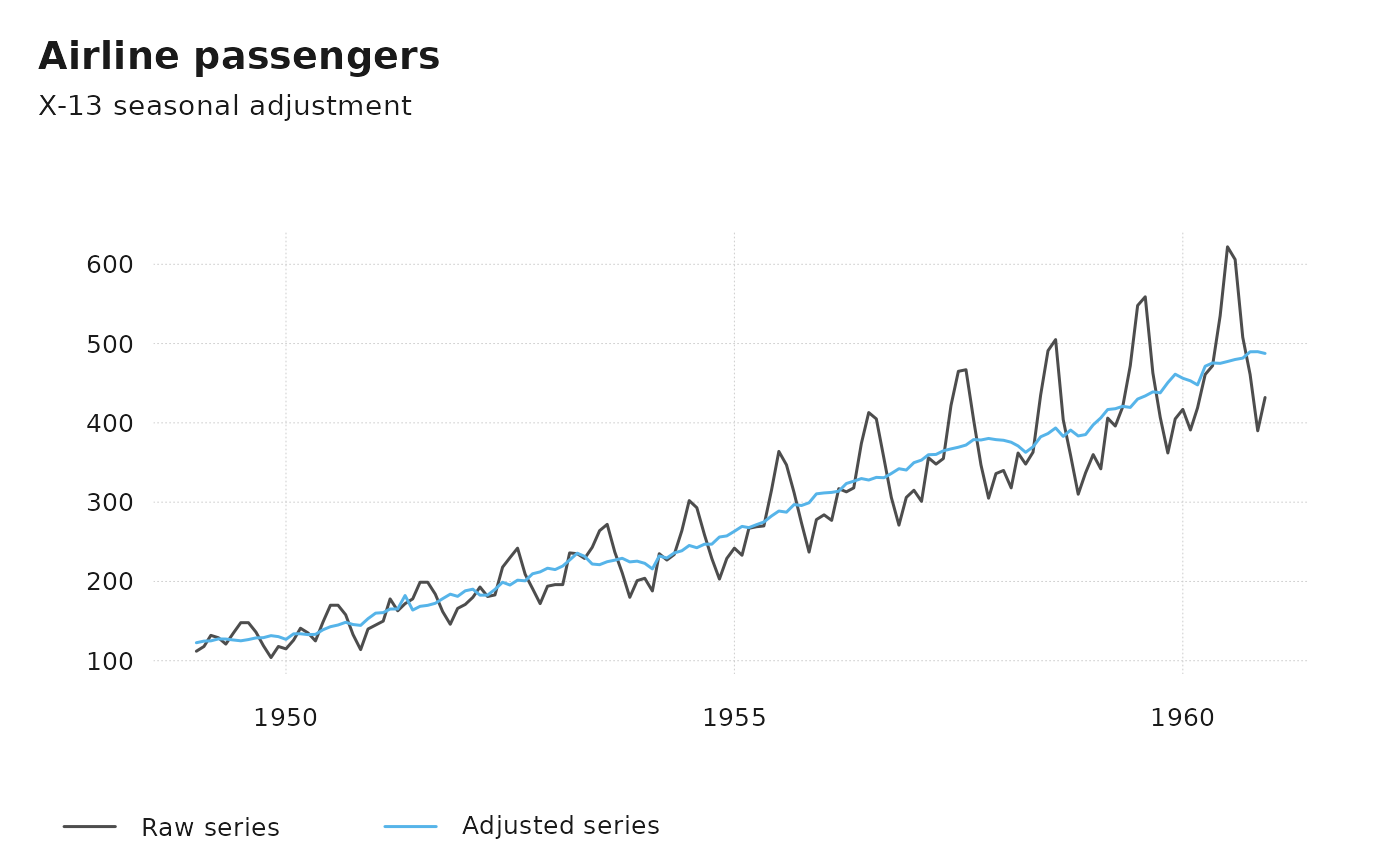Example Functions, Generated by ts_. `ts_prcomp` calculates the principal components of multiple time series, `ts_dygraphs` generates an interactive graphical visualization, `ts_forecast` return an univariate forecast, `ts_seas` the seasonally adjusted series. `ts_na_interpolation` imputes missing values.

## Usage

``````ts_prcomp(x, ...)

ts_dygraphs(x, ...)

ts_forecast(x, ...)

ts_seas(x, ...)

ts_na_interpolation(x, ...)``````

## Arguments

x

ts-boxable time series, an object of class `ts`, `xts`, `zoo`, `zooreg`, `data.frame`, `data.table`, `tbl`, `tbl_ts`, `tbl_time`, `tis`, `irts` or `timeSeries`.

...

further arguments, passed to the underlying function. For help, consider these functions, e.g., stats::prcomp.

## Value

a ts-boxable object of the same class as `x`, i.e., an object of class `ts`, `xts`, `zoo`, `zooreg`, `data.frame`, `data.table`, `tbl`, `tbl_ts`, `tbl_time`, `tis`, `irts` or `timeSeries`.

## Details

With the exception of `ts_prcomp`, these functions depend on external packages.

Vignette on how to make arbitrary functions ts-boxable.

## Examples

``````# \donttest{
ts_plot(
ts_scale(ts_c(
Male = mdeaths,
Female = fdeaths,
`First principal compenent` = -ts_prcomp(ts_c(mdeaths, fdeaths))[, 1]
)),
title = "Deaths from lung diseases",
subtitle = "Normalized values"
)ts_plot(ts_c(
male = mdeaths, female = fdeaths,
ts_forecast(ts_c(`male (fct)` = mdeaths, `female (fct)` = fdeaths))
),
title = "Deaths from lung diseases",
subtitle = "Exponential smoothing forecast"
)
#> Registered S3 method overwritten by 'quantmod':
#>   method            from
#>   as.zoo.data.frame zoots_plot(
`Raw series` = AirPassengers,
title = "Airline passengers",
)# See ?imputeTS::na_interpolation for options
dta <- ts_c(mdeaths, fdeaths)
dta[c(1, 3, 10), c(1, 2)] <- NA